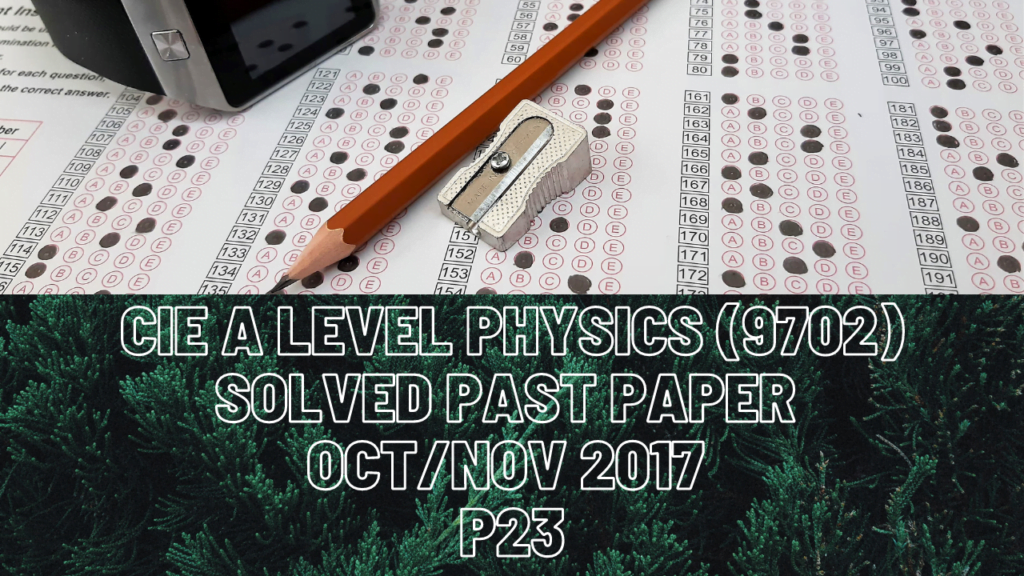A Level Physics### CIE A Level Physics Solved Past Paper Oct/Nov 2017 P23

1 bii) P=kAT^4 can be written in the form y=constant*x^2 because x-axis is x^2 and y-axis is P so a graph of y=x^2 is always an increasing gradient graph with positive slope.

2 bi) Since the solid sphere is moving with constant velocity, all forces acting on it in different directions must be balanced.

ci) The pressure at the surface of any liquid (when x=0.17m above the base of container) is due to atmosphere.

3 bi) The total momentum before collision along the y-axis is zero and that after the collision is due to ball A that moves upwards and ball B which moves down so it will have its momentum with negative magnitude.

ii) The total momentum of balls A and B before the collision along the x-axis will be equal to that after the collision. Total momentum before the collision is all due to ball A and is 4v and that after the collision is due to both balls since they both are moving in the same direction along the x-axis after head-on collision. Ball A moves with momentum 4*(6cos(theta)) towards the right and ball B moves with 12*(3.5cos(35)) of momentum towards the right. YOU HAVE TO BE VERY CAREFUL WITH SIGNS WHEN DEALING WITH MOMENTUM CONSERVATION.

iii) If kinetic energy is not conserved during collision then that collision is not elastic. Total momentum is always conserved regardless of whether a collision is elastic or inelastic.

4 ci) Point Y is nearer to the observer standing at point O than point X is so intensity or amplitude of sound heard from point Y is going to be a lot higher than that from point X.

ii) The car moves from X to Y so frequency of sound heard from point Y is going to be higher. Relationship between frequency and wavelength is inverse proportionality so a higher frequency observed from point Y means sound has its minimum wavelength at point Y.

5c) Although force due to electric field on alpha particle is double the force on the proton, its mass is also 4 times higher thus it accelerates at half a rate than a proton does.

6d) There will be no effect on current that flows through filament wire (because no change to the charge) but resistance will be a lot less this time when temperature falls with decrease in p.d. since resistance is directly proportional to temperature.